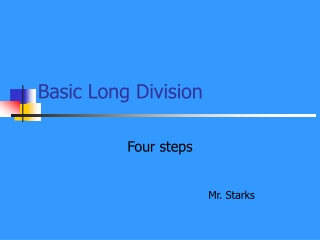DownloadDownload PresentationBasic Long Division

# Basic Long Division

Download Presentation## Basic Long Division

- - - - - - - - - - - - - - - - - - - - - - - - - - - E N D - - - - - - - - - - - - - - - - - - - - - - - - - - -
##### Presentation Transcript

1. Basic Long Division Four steps Mr. Starks

2. The Four Steps Are: • Divide • Multiply • Subtract • Bring Down

3. Step One--Divide Divide the divisor into the dividend---this creates a quotient. quotient 3 divisor 2 7 dividend

4. Step Two--Multiply Multiply the quotient times the divisor.Write it under the dividend. quotient 3 divisor 2 7 dividend 6

5. Step Three--Subtract Subtract the number under the dividend from it. quotient 3 divisor 2 7 dividend 6 1

6. Step Four—Bring Down Bring down the next dividend and start the process again. quotient 3 divisor 2 7 2 dividend 6 1 2